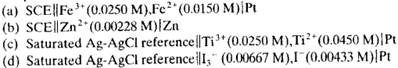### Create an Account

Home / Questions / Calculate the theoretical potential of the following cells n each case assume that activit...

# Calculate the theoretical potential of the following cells n each case assume that activities are approximately equal to molar concentrations and that the temperature is 25°C

Calculate the theoretical potential of the following cells. ([n each case assume that activities are approximately equal to molar concentrations and that the temperature is 25°C.)Jun 23 2020 View more View LessSubscribe To Get Solution